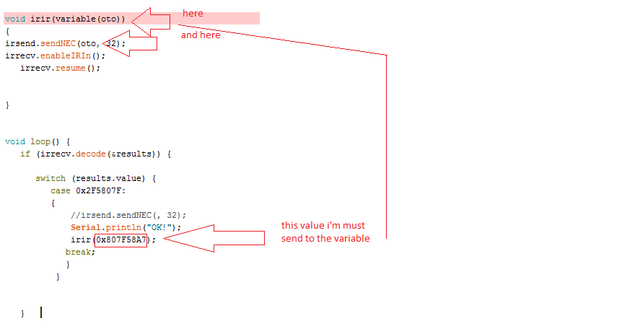# How to pass the hex value to a variable in another void

Hello, how to pass the hex value to a variable in another procedure (void)

My code

``````#include <IRremote.h>
#include <IRremoteInt.h>
int RECV_PIN = 11;
byte ircom;
IRrecv irrecv(RECV_PIN);
decode_results results;
IRsend irsend;
void setup()
{
Serial.begin(9600);
}

void irir(variable(oto)) //<-- here
{
irsend.sendNEC(oto, 32);
irrecv.enableIRIn();
irrecv.resume();

Serial.println(oto);
}

void loop() {
if (irrecv.decode(&results)) {

switch (results.value) {
case 0x2F5807F:
{
//irsend.sendNEC(, 32);
Serial.println("OK!");
irir(0x807F58A7); //<--- this value
break;
}
}

}

}
``````
``````Serial.begin(9600);
``````

Here, you are passing the hex value 0x2580 to a function.

`variable(oto)` is "unsigned long oto"

I do not understand why I should use the serial port to pass values to a variable in another procedure. I think I explained what I mean in the picture.
Regards``````void irir(variable(oto)) //<-- here
``````

Try:

``````void irir(unsigned long oto) {
``````
``````Serial.begin(9600);
``````

Is an example of passing a value to a function. In this case a decimal value

The point is that it is exactly the same as doing

``````Serial.begin(0x2580);
``````

Hex is only one of many ways to represent a number

The serial was just an example. Your code looks OK at first glance.

If you don't want to pass a hard-coded value, you can pass results.value in the function call.

jremington:

``````void irir(variable(oto)) //<-- here
``````

Try:

``````void irir(unsigned long oto) {
``````

Thank you, also works.

Why my arduino frezzing after few uses.

``````#include <IRremote.h>
#include <IRremoteInt.h>
int RECV_PIN = 11;
long ot = 0x0000000;
IRrecv irrecv(RECV_PIN);
decode_results results;
IRsend irsend;
void setup()
{
}

void irir(unsigned long oto)
{
irsend.sendNEC(oto, 32);
irrecv.enableIRIn();
delay(500);

}

void loop() {

if (irrecv.decode(&results)) {
ot=results.value;
switch (ot) {
case 0x2F5807F: {  irir(0x807F58A7); break; }
case 0x2F5C03F: {  irir(0x807FE817); break; }
case 0x2F540BF: {  irir(0x5FA38C7); break; }
case 0x2F59867: {  irir(0xE8AFBC77); break; }
case 0x2F548B7: {  irir(0x807F42BD); break; }
case 0x2F5A05F: {  irir(0x807FB04F); break; }
case 0x2F57887: {  irir(0x5FABA45); break; }
case 0x2F5F00F: {  irir(0x5FA02FD); break; }

}
}

}
``````

No resume?

After a few uses, it hangs and does not resume, it also happens as I hold the button on the remote control.

You don't have a call to resume (that I can see)